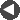Winsock Programmer's FAQ Example: How to Pass a Socket HandleThis example is based on the example in the Microsoft documentation for the _pipe() run-time library function. It was brought to my attention by Valerie Puffet-Michel. I took the example and made it a bit more robust, partly to catch the Borland C++ bug described below.

Essentially, this example works on the Win32 rule that a child process can inherit the file handles that the parent opened. The program passes the file handle IDs on the command line to the child that it spawns. Since file handles and socket descriptors are the same thing in Win32, this works just as well for sockets as it does for the pipe handles used in the example.

#### fdpass.cpp

```// Borland C++ 5.0: bcc32 fdpass.cpp
// Visual C++ 5.0:  cl fdpass.cpp

#include <stdlib.h>
#include <stdio.h>
#include <io.h>
#include <fcntl.h>
#include <process.h>
#include <math.h>

enum PIPES {
WRITE = 1
};

#define NUMPROBLEM 8

#ifdef _MSC_VER
#define CWAIT _cwait
#else
#define CWAIT cwait
#endif

int main(int argc, char *argv[])
{
int hpipe;
char hstr;
int pid, problem, c;
int termstat;

if (argc == 1) {
//// No arguments, so this must be the parent process
// Open a set of pipes
if (_pipe(hpipe, 256, O_BINARY) == -1) {
perror("pipe failed");
exit(1);
}

// Convert read side of pipe to string and pass as an argument
// to the child process.
if ((pid = spawnl(P_NOWAIT, argv, argv, hstr, NULL)) == -1) {
perror("Spawn failed");
}

// Put problem in write pipe; it will appear in child's read pipe.
for (problem = 1000; problem <= NUMPROBLEM * 1000; problem += 1000) {
if (write(hpipe[WRITE], (char *) &problem, sizeof(int)) == -1) {
perror("parent write failed");
}
else {
printf("Son, what is the square root of %d?\n", problem);
}
}

// Wait until spawned program is done processing.
CWAIT(&termstat, pid, WAIT_CHILD);
if (termstat & 0x0) {
perror("Child failed");
}

close(hpipe[WRITE]);
}
else {
//// There is a command line argument, so we must be a child process
// Convert argument to integer handle value.

// Read problem from pipe and calculate solution.
for (c = 0; c < NUMPROBLEM; c++) {
}
else {
printf("Dad, the square root of %d is %3.2f.\n", problem,
sqrt((double) problem));
}
}
}

return 0;
}
```

 << Ping: Raw Sockets Method Get the Local IP Address(es) >> Last modified on 6 October 2001 at 13:04 UTC-7 Please send corrections to tangent@cyberport.com.
 < Go to the main FAQ page << Go to the Home Page